Science, Maths & Technology

### Become an OU student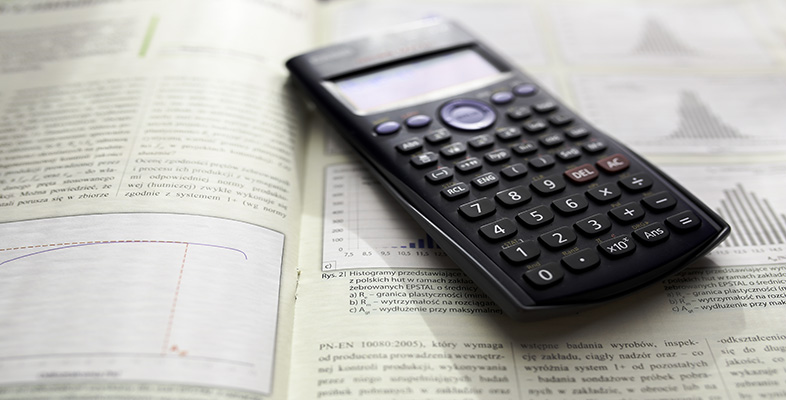Using a scientific calculator

Start this free course now. Just create an account and sign in. Enrol and complete the course for a free statement of participation or digital badge if available.

# 1.2 Fractions or decimals?

In Activity 1 you set up your calculator to use Math mode. In this mode, when the result of a calculation is not a whole number, it will be displayed as a fraction, such as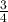, wherever possible.

To obtain the answer in decimal form, you need to press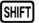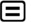instead of, or you can toggle between the fractional and decimal outputs using the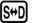key.

Remember, your calculator is in Math mode if the word Math is shown at the top of the calculator display. If your calculator is not in Math mode, repeat the steps of Activity 1.

## Activity 3 Fractions and decimals

Use your calculator to find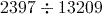in both fractional and decimal forms.

In Math mode, calculatinggives the result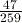.
Converting this to a decimal usinggives 0.1814671815.